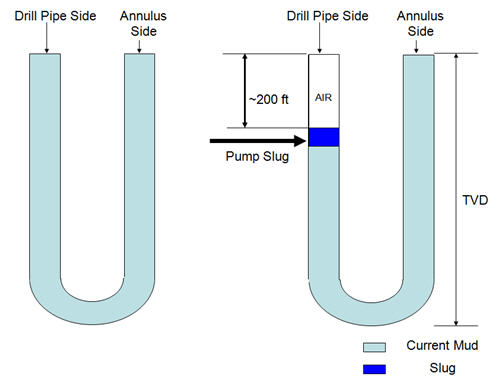# Barrels of slug required for desired length of dry pipe

What is slug? Slug: It is heavy mud which is used to push lighter mud weight down before pulling drill pipe out of hole. Slug is used when pipe became wet while pulling out of hole. This article will demonstrate you how to calculate how many barrel of volume slug required for desired light of dry pipe.

Normally, 1.5 to 2 PPG over current mud weight is a rule of thumb to decide how much weight of slug should be. For example, current mud weight is 10 PPG. Slug weight should be about 11.5 to 12 PPG.  Generally, slug is pumped to push mud down approximate 200 ft and slug volume can be calculated by applying a concept of U-tube (See Figure below).

Volume of slug required for required length of dry pipe can be calculated by this following equations:

## Oilfield Unit

Step 1: Determine hydrostatic pressure required to give desired drop inside drill pipe:

Hydrostatic Pressure in psi = mud weight in ppg × 0.052 × ft of dry pipe

Step 2: Determine difference in pressure gradient between slug weight and mud weight:

Pressure gradient difference in psi/ft = (slug weight in ppg – mud weight in ppg) × 0.052

Step 3: Determine length of slug in drill pipe:

Slug length in ft = Hydrostatic Pressure in psi (in step 1) ÷ Pressure gradient difference in psi/ft (step 2)

Step 4: Slug volume required in barrels:

Slug volume in barrel = Slug length in ft × drill pipe capacity in bbl/ft

Example: Determine the barrels of slug required for the following:

Desired length of dry pipe = 200 ft

Drill pipe capacity = 0.016 bbl/ft

Mud weight = 10.0 ppg

Slug weight = 11.5 ppgStep 1 Hydrostatic pressure required:

Hydrostatic Pressure in psi = 10.0 ppg × 0.052 × 200 ft

Hydrostatic Pressure in psi = 104 psi

Step 2 differences in pressure gradient between slug weight and mud weight:

Pressure gradient difference in psi/ft = (11.5 ppg – 10.0 ppg) × 0.052

Pressure gradient difference in psi/ft = 0.078 psi/ft

Step 3 length of slug in drill pipe:

Slug length in ft = 104 psi ÷ 0.078 psi/ft

Slug length in ft = 1,333 ft

Step 4 Slug volume required in barrels:

Slug volume required = 1,333 ft × 0.016 bbl/ft

Slug volume required = 21.3 bbl

## Metric Unit

Step 1: Determine hydrostatic pressure required to give desired drop inside drill pipe:

Hydrostatic Pressure in kPa = mud weight in kg/m³ × 0.00981 × length of dry pipe in m

Step 2: Determine difference in pressure gradient between slug weight and mud weight:

Pressure gradient difference in kPa/m = (slug weight in kg/m³ – mud weight in kg/m³) × 0.00981

Step 3: Determine length of slug in drill pipe:

Slug length in m = Hydrostatic Pressure in kPa (in step 1) ÷ Pressure gradient difference in kPa/m(step 2)

Step 4: Slug volume required in barrels:

Slug volume in m³ = Slug length in m × drill pipe capacity in m³/m

Example: Determine the barrels of slug required for the following:

Desired length of dry pipe = 120 m

Drill pipe capacity = 0.00782 m³/m

Mud weight = 1,380 kg/m³

Slug weight = 1,500 kg/m³

Step 1 Hydrostatic pressure required:

Hydrostatic Pressure in psi = 1,380 kg/m³ × 0.00981 × 120 m

Hydrostatic Pressure in psi = 1,625 kPa

Step 2 differences in pressure gradient between slug weight and mud weight:

Pressure gradient difference in kPa/m = (1,500 kg/m³ – 1,380 kg/m³ ) × 0.00981

Pressure gradient difference in kPa/m = 1.1772 kPa/m

Step 3 length of slug in drill pipe:

Slug length in ft = 1,625 kPa ÷ 1.1772 kPa/m

Slug length in ft = 1,380 m

Step 4 Slug volume required in barrels:

Slug volume required = 1,380 m × 0.00782 m³/m

Slug volume required = 10.79 m³

Ref books:

Lapeyrouse, N.J., 2002. Formulas and calculations for drilling, production and workover, Boston: Gulf Professional publishing.

Bourgoyne, A.J.T., Chenevert , M.E. & Millheim, K.K., 1986. SPE Textbook Series, Volume 2: Applied Drilling Engineering, Society of Petroleum Engineers.

Mitchell, R.F., Miska, S. & Aadny, B.S., 2011. Fundamentals of drilling engineering, Richardson, TX: Society of Petroleum Engineers.

Share the joyWorking in the oil field and loving to share knowledge.

### 12 Responses to Barrels of slug required for desired length of dry pipe

1.Jim Pohl says:

For what reason is slug mud used to produce dry pipe?

•DrillingFormulas.Com says:

To keep drill pipe dry while pulling out of hole.

2.Almaz says:

Can the slug keep the pipe dry if there is a mud motor in the BHA?

•DrillingFormulas.Com says:

Yes. If you pump enough volume and weight, the slug will keep the pipe dry even though you have mud motor in the drill string.

3.Almaz says:

thank you. how different is the calculation of slug volume if there is a PDM?

•DrillingFormulas.Com says:

No different in calculation even though you have the slug in the PDM.

4.Emiro says:

Which are the steps to pumping a slug? (the number of strokes)

•DrillingFormulas.Com says:

You can follow the steps below for pumping slug.

1. Pump slug as per your volume required.
2. Pump volume of mud equal to surface line to chase up the slug
3. Let slug fall
4. Flow check to ensure that slug stops fall into the drill string.

5.bibka says:

how can know % of return when drilling ( mud i mean )

•DrillingFormulas.Com says:

% return is tracked by flow show.

6.Rafael says:

Hey, nice explanation, but at step 2, u used mud 10.5ppg instead of 10ppg.
Ty

•DrillingFormulas.Com says:

Rafael,

Thanks for this. I correct my typo mistake already.

Regards,
Shyne.

This site uses Akismet to reduce spam. Learn how your comment data is processed.